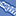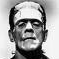# QlikView App Dev

Discussion Board for collaboration related to QlikView App Development.

Announcements
Join us at the Cloud Data and Analytics Tour! REGISTER TODAY
cancel
Showing results for
Search instead for
Did you mean:Creator

## Help with number in text

Hi, could anyone help me get fix the number in the text?

below is my formula:

='2017 ('& ' \$(=\$(Max_Clos_2017))' & '+' & (12- '\$(=\$(Max_Clos_2017))')    & ')' &     '= R\$ '&

(Sum(Aggr(

(Sum(DISTINCT(if((left(Month,2)<= ('\$(=\$(Max_Clos_2017))'))and ('\$(=\$(Max_Clos_2017))')=Currency_Closing_Period ,Act_Rate))))

+

(Sum(DISTINCT(if((left(Month,2)> ('\$(=\$(Max_Clos_2017))')) and ('\$(=\$(Max_Clos_2017))')=Currency_Closing_Period ,Est_Rate))))

,Month)))/12

the result is: 2017 ( 5+7)= R\$ 3,215375. what change i should make so that the number would be 3,2153 only?

thanks

4 RepliesMaster II

Round(

Your Number Expression ,.0001)MVP

May be this

='2017 ('& ' \$(=\$(Max_Clos_2017))' & '+' & (12- '\$(=\$(Max_Clos_2017))')    & ')' &     '= R\$ '&

Floor((Sum(Aggr(

(Sum(DISTINCT(if((left(Month,2)<= ('\$(=\$(Max_Clos_2017))'))and ('\$(=\$(Max_Clos_2017))')=Currency_Closing_Period ,Act_Rate))))

+

(Sum(DISTINCT(if((left(Month,2)> ('\$(=\$(Max_Clos_2017))')) and ('\$(=\$(Max_Clos_2017))')=Currency_Closing_Period ,Est_Rate))))

,Month)))/12, 0.0001)Creator
Author

totally worked! thanksMaster

Hi Silvia,

Please remember to mark this question as Answered by Sunny.

Kind regards

Andrew